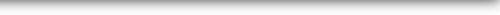DASP

Distributive Analysis Stata PackageDASP Modules

## Inequality

ICVAR Estimates the coefficient of variation and their standard errors taking full account of survey design.

DICVAR Estimates the difference between coefficient of variation indices and its standard error taking full account of survey design.

## Polarisation

DSPOL To estimate the Inaki (2008) social polarisation index

## Poverty

ISST Estimates the Sen-Shorrocks-Thon index of poverty and their standard errors taking full account of survey design.

DISST Estimates the difference Sen-Shorrocks-Thon  indices and its standard error taking full account of survey design.

IWATTS Estimates the Watts index of poverty and their standard errors taking full account of survey design.

DIWATTS Estimates the difference between Watts indices of poverty and its standard error taking full account of survey design.

## Decomposition

RBDINEQ

The command rbdineq use the regression based decomposition approach to decompose inequality indices by income covariates.
The decomposition is performed using the Shapley value or the analytical approach. With the Shapley approach, the user can
select among the following inequality indices:

. Gini
. Atkinson
. Coefficient of variation
. Generalised entropy

DSINEQS

Decomposes inequality indices by income sources with the Shapley approach (Gini/Atkinson/Generalised Entropy/Coefficient of variantion).

## Curves

CPROG

The module cprog produces the progressivity curves (PR(p)) for a given list of variables (components).

Let x be a gross income.
. A tax t is Tax Redistribution (TR) progressive if:
. PR(p) = L_x(p) - C_t(p) > 0 for all p in ]0, 1[

. A transfer b is Tax Redistribution (TR) progressive if:
. PR(p) = C_b(p) - L_x(p) > 0 for all p in ]0, 1[

. A tax t is Income Redistribution (IR) progressive if:
. PR(p) = C_x-t(p) - L_x(p) > 0 for all p in ]0, 1[

. A transfer b is Income Redistribution (IR) progressive
. PR(p) = C_x+b(p) - L_x(p) > 0 for all p in ]0, 1[

CPROGBT

The module cprogbt produces the progressivity curves to check if the tranfer b is more progressive that the tax t.(components).

Let x be a gross income.
. A transfer B is more Tax Redistribution (TR) than the tax T if:
. PR(p) = C_b(p) + C_t(p)- 2L_x(p) > 0 for all p in ]0, 1[

. A transfer B is more Income Redistribution (IR) than the tax T if:
. PR(p) = C_x+b(p) - C_x-t(p) > 0 for all p in ]0, 1[

## Distributive tools

IMEAN Estimates the statistic average -mean- and their standard errors taking full account of survey design.

DIMEAN Estimates the difference between averages -means-  and its standard error taking full account of survey design.